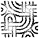# Progressive Rotate

The CDC-6000 series has instructions for testing the high-order (sign) bit of a register but no other bit addressing. Therefore:Test bits by rotating them into the sign position. All rotates are equally fast so bits can be tested in any order. A register need not be restored to its original positon between tests so long as the current postion of the register is known before each test. In complicated test sequences additional rotates may be added to balance the paths.
Use the instruction sequence:
LXi   a-b
PL    Xi,label
where a is the bit number currently in the sign postion and b is the bit number of the bit desired there. Caution, CDC convention numbers bits from 0 to 59 starting at the least significant bit, while DEC convention numbers the PDP-8's bits from 0 to 11 starting at the most significant bit. CDC code is written using the CDC convention.

PDP-8 Simulator